# The robustness of the tensor product

 Importance: High ✭✭✭
 Subject: Theoretical Computer Science » Coding Theory
 Keywords: codes coding locally testable robustness
 Posted by: ormeir on: July 13th, 2007
Problem   Given two codes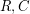, their Tensor Productis the code that consists of the matrices whose rows are codewords ofand whose columns are codewords of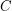. The productis said to be robust if whenever a matrixis far from, the rows (columns) ofare far from(, respectively).

The problem is to give a characterization of the pairswhose tensor product is robust.

The question is studied in the context of Locally Testable Codes.

## Bibliography

*[BS] Eli Ben-Sasson, Madhu Sudan, Robust locally testable codes and products of codes, APPROX-RANDOM 2004, pp. 286-297 (See ECCC TR04-046).

[CR] D. Coppersmith and A. Rudra, On the robust testability of tensor products of codes, ECCC TR07-061.

[DSW] Irit Dinur, Madhu Sudan and Avi Wigderson, Robust local testability of tensor products of LDPC codes, APPROX-RANDOM 2006, pp. 304-315 (See ECCC TR06-118).

[GM] Oded Goldreich, Or Meir, The Tensor Product of Two Good Codes Is Not Necessarily Robustly Testable, ECCC TR07-062.

[M] Or Meir, On the Rectangle Method in proofs of Robustness of Tensor Products, ECCC TR07-061.

[V] Paul Valiant, The Tensor Product of Two Codes Is Not Necessarily Robustly Testable, APPROX-RANDOM 2005, pp. 472-481.

* indicates original appearance(s) of problem.

### Results in

Eli Ben-Sasson and Michael Viderman. "Composition of semi-LTCs by two-wise Tensor Products" (RANDOM 09)

Eli Ben-Sasson and Michael Viderman. "Tensor Products of Weakly Smooth Codes are Robust" (RANDOM 08)

### The formal definition of robustness, and of the problem

In all of the following definitions, the term "distance" refers to "relative Hamming distance".

Given a matrix, let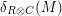denote the distance fromto the nearest codeword of. Letdenote the average distance of a row ofto, and letdenote the average distance of a column ofto. Finally, let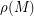denote the average ofand.

The tensor productis said to be-robust iff for every matrixwe have that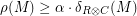.

The question is, under what conditions the tensor productis-robust for some constant.

### To be more precise ...

1) When you say codes, do you mean linear codes?

2) What distance you are using when you're saying "far from"?

### To be more precise

1) The question is most interesting for linear codes, but it can also be defined for non-linear codes.

2) The distance is (relative or absolute) Hamming Distance.